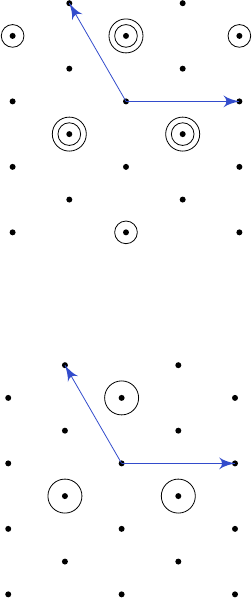6Representation of Lie algebras

III Symmetries, Fields and Particles6.4 Decomposition of tensor products
The last thing we need to know how to do is how we can decompose tensor
products of irreps. This is not hard. It is just what we did for
su
(2) but in a
more general context.
We let
ρ
Λ
and
ρ
Λ
0
be irreps of
g
with corresponding representation spaces
V
Λ
and V
0
Λ
0
. Then we can write
V
Λ
=
M
λS
Λ
V
λ
, V
0
Λ
0
=
M
λ
0
S
Λ
0
V
0
λ
0
.
If v
λ
V
Λ
and v
λ
0
V
0
Λ
0
, then for H h, we have
(ρ
Λ
ρ
Λ
0
)(H)(v
λ
v
λ
0
) = ρ
Λ
(H)v
λ
v
λ
0
+ v
λ
ρ
Λ
0
(H)v
λ
0
= λ(H)v
λ
v
λ
0
+ λ
0
(H)v
λ
v
λ
0
= (λ + λ
0
)(H)v
λ
v
λ
0
.
Since things of the form v
λ
v
λ
0
span V
Λ
V
Λ
0
, we know
S
ΛΛ
0
= {λ + λ
0
: λ S
Λ
, λ
0
S
Λ
0
}
with multiplicities. To figure out the decomposition, we find out what the highest
weight of the tensor product is, and then subtract off the factors corresponding
to the highest weight, and then repeat.
Example.
Consider
g
=
A
2
, and the fundamental representation
ρ
(1,0)
. We
consider
ρ
(1,0)
ρ
(1,0)
.
Recall that the weight set is given by
S
(1,0)
= {ω
(1)
, ω
(1)
+ ω
(2)
, ω
(2)
}.
We can then draw the collection of all weights in ρ
(1,0)
ρ
(1,0)
as follows:
α
β
So we see that we have a highest weight of (2
,
0). We then use the algorithm to
compute all the weights of such an irrep, remove it from the weight lattice, and
be left with
α
β
This is just the anti-fundamental representation. So we have
3 3 = 6
¯
3.